# COUNTA Excel FunctionArticle byExcelMojo Team## What Is COUNTA Excel Function?

The COUNTA Excel Function in Excel calculates all non-blank cells containing numerical and alphabetical values in a table array. It is a Statistical Excel Function and returns only a numerical value.

For example, in the following image, we will count all the non-blank cells having numbers and alphabets in column A using the COUNTA Excel function in cell B2.

=COUNTA(A2:A5)

The output comes as ‘3’.

###### Key Takeaways
• The COUNTA formula enables users to count the cells containing numeric values, text values, cell references, ranges, date, time, and error values in Excel. It is a Statistical Excel Function.
• The syntax of the COUNTA function is =COUNTA(value1,[value2],…). Here, value1 is a mandatory argument, and value2 is an optional argument. They are the values to be counted.
• The COUNTA function does not count on an empty table array and returns the output as 0.
• The COUNTA function has a hidden feature, i.e., the empty string within quotes (“ “) is passed as an argument is also counted.

### COUNTA() Excel Syntax

The image below is an explanation of the arguments accepted by the COUNTA function in Excel:

• value1 = The first cell reference or the range we want to count. It is the mandatory argument.
• value2 = The second cell reference in excel or the range we want to count. It is the optional argument.

### How To Use COUNTA Function?

We can use the COUNTA function in Excel in two ways:

1. Choose the empty cell which will contain the result.
2. Go to the “Formulas” tab and click it.
3. Select “More Functions” from the drop-down menu.
4. Click on “Statistical” from the drop-down menu.
5. Select “COUNTA” from the drop-down menu.
6. A window called “Function Arguments”
7. As the number of arguments, enter the value in the “Value 1” and “Value 2”.
8. Select OK.

#### 2. Manually On The Sheet

• Select the empty cell
• Type =COUNTA(
• Double click on the COUNTA command
• Select the range of the cell or array
• Press “Enter.”

The image below depicts values in various formats, including numbers, decimal numbers, numbers in percentage, letters, fruit names, prices with the ‘\$’ sign, and no text string within (“ “) quotes. Here, we will try to calculate the number of all non-blank cells using the COUNTA function.

In the table, the data is reflected as below:

• Column A shows values in various formats

The steps to count the number of non-blank cells are as follows:

1. First, we will choose the cell where we want the result to show up. Cell B2 would be the cell in this case.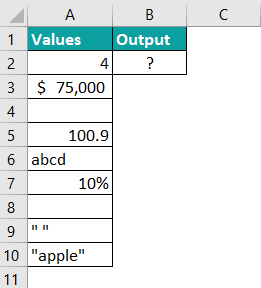2. Enter the formula to count values from the table. Select the value1, which is the range from the starting cell address to the ending cell address of the table, i.e., A2:A10. The complete COUNTA Excel function will be:

=COUNTA(A2:A10)3. After entering each value in the preceding step, press the “Enter” key. The result is shown in cell B2 as ‘7’ in the image below.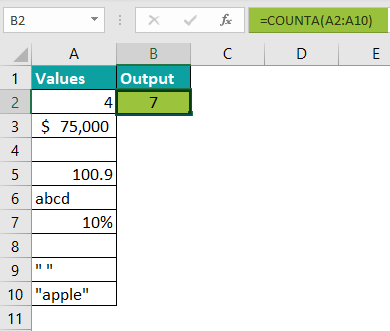Therefore, the COUNTA function counts all the numbers, decimal numbers, numbers in percentage, letters, fruit name, price with the ‘\$’ sign, and no text string within (“ “) quotes but does not count the blank spaces in the table. For example, the total cells in the table are 9, but there are two empty cells, which the COUNTA function did not count.

### Examples

Let us look at some advanced COUNTA Excel function examples to understand how it works:

#### Example #1 – Continuous Rectangular Range

The image below depicts the data of people working in a firm from different locations. The values include their ID, Date of joining, Location, Age, and Gender. Here, we will try to calculate the number of all cells having numbers and letters using the COUNTA function.

In the table, the data is reflected as below:

• Column A shows ID
• Column B contains the Date
• Column C contains the Location
• Column D contains the Age
• Column E contains Gender

The steps to count the number of non-blank cells are as follows:

Step 1:  First, we will choose the cell where we want the result to show up. Cell E7 would be the cell in this case.

Step 2: Enter the formula to count cells with values from the table. Select the value1, which is the range from the starting cell address to the ending cell address of the table, i.e., A2:E6. The complete COUNTA Excel function will be:

=COUNTA(A2:E6)

Step 3: After entering each value in the preceding step, press the “Enter” key. The results are shown in cell E7 as ‘22’ in the image below.

Therefore, the COUNTA function counts all the numbers, words, and dates but does not count the blank spaces in the table. For example, the total cells in the table are 25, but there are three empty cells in the table, which the COUNTA function did not count.

#### Example #2

The image below depicts the data of fruit vendors. The values include the fruits and their prices. Here, we will try to calculate the number of all cells having numbers and letters using the COUNTA function.

In the table, the data is reflected as below:

• Column A shows Fruits
• Column B contains the Price

The steps to count the number of non-blank cells are as follows:

Step 1:  First, we will choose the cell where we want the result to show up. Cell C2 would be the cell in this case.

Step 2: Enter the formula to count cells with values from the table. Select the value1, which is the range from the starting cell address to the ending cell address of the table, i.e., A2:B10. The complete COUNTA Excel function will be:

=COUNTA(A2:B10)

Step 3: After entering each value in the preceding step, press the “Enter” key. The result is shown in cell C2 as ‘13’ in the image below.

Therefore, the COUNTA function counts all the numbers and words but does not count the blank spaces in the table. For example, the total cells in the table are 18, but there are five empty cells, which the COUNTA function did not count.

#### Example #3 – Return 0

The COUNTA function typically counts non-blank cells in an array. However, the image below depicts no value. Therefore, we will try to calculate the empty cells having no value using the COUNTA function.

In the table, the data is reflected as below:

• Column A shows a blank cell

The steps to count the number of non-blank cells are as follows:

Step 1:  First, we will choose the cell where we want the result to show up. Cell B2 would be the cell in this case.

Step 2:   Enter the formula that needs to count values from the table. Select the value1, the cell from the table, i.e., “A2”. The complete COUNTA Excel function will be:

=COUNTA(A2)

Step 3: After entering the value in the preceding step, press the “Enter” key. The result in cell B2 is ‘0’ in the image below.

Therefore, the COUNTA function counts all the cells with values but does not count the blank spaces in the table. As a result, the table has no value, so the COUNTA function returns 0 as the output.

### Important Things To Note

• The COUNTA function does not count blank cells.
• It simply counts the existing values rather than adding them up.
• If the arguments are invalid, it returns an error.
• The COUNTA function is part of the COUNT excel function group.
• COUNTA function counts text values and numeric values.
• The function can handle up to 255 characters.

How to do COUNTA in Excel?

Below is the step by step process of counting non-blank cells using the COUNTA function:
1. Select the empty cell
2. Type =COUNTA(
3. Double click on the COUNTA command
4. Select the range of the cell or array
5. Press “Enter.”

For example, the image below shows the Annual Budget of the firm’s Departments. The values include the Departments and the amount of the Annual Budget. Here, we will try to calculate the number of all cells having numbers and letters and no text String within (“ “) quotes using the COUNTA function.

In the table, the data is reflected as below:
– Column A shows the Departments
– Column B contains the Annual Budget
– Cell C2 calculates the number of numeric value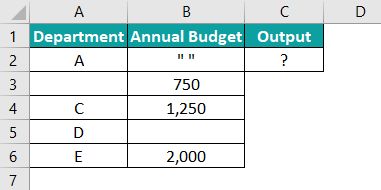Enter the COUNTA Excel function in Cell C2.
=COUNTA(A2:B6)Press the “Enter” key. The results are shown in cell C2 as ‘8’ of the image below.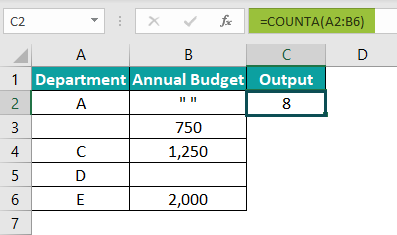Therefore, the COUNTA function counts the number and letters and no text string within (“ “) quotes as a value.

Where is the COUNTA function in Excel?

One can activate the COUNTA function in Excel using the following steps:
1. Choose the empty cell which will contain the result.
2. Go to the “Formulas” tab and click it.
3. Select “More Functions” from the drop-down menu.
4. Select “Statistical” from the drop-down menu.
5. Select “COUNTA” from the drop-down menu.
6. A window called “Function Arguments” appears.
7. As the number of arguments, enter the value in the “Value 1” and “Value 2”
8. Select OK.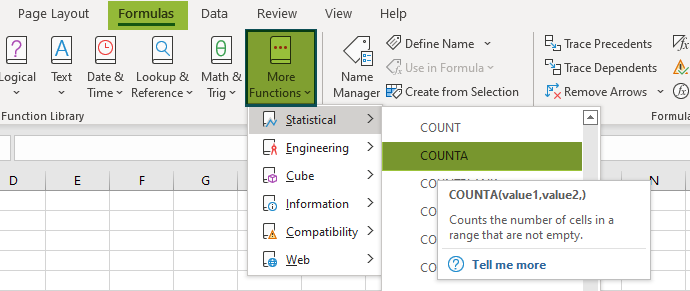How to COUNTA function in Excel with multiple criteria?

The image below depicts the mixed content. The values include the letters, phrases, numbers, dates, and empty text strings within (“ “) quotes. Here, we will try to calculate the number of all cells having content except blank cells with multiple criteria, i.e., passing multiple arguments using the COUNTA function.

In the table, the data is reflected as below:
– Columns A and B contain Contents

The steps to count the number of non-blank cells are as follows:

Step 1:  First, we will choose the cell where we want the result to show up. Cell B7 would be the cell in this case.Step 2:   Enter the formula that needs to count values from the table. Select the value1, which is the range from the starting cell address to the ending cell address of the table, i.e., A2:A6.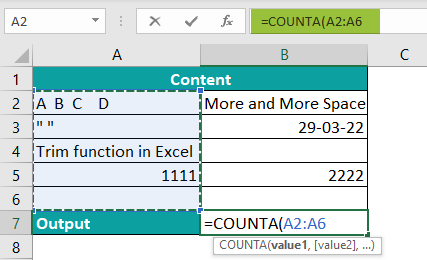Step 3: Select the value2, which ranges from the starting cell address to the ending cell address of the table, i.e., B2:B6. The complete COUNTA Excel function will be:
=COUNTA(A2:A10,B2:B6)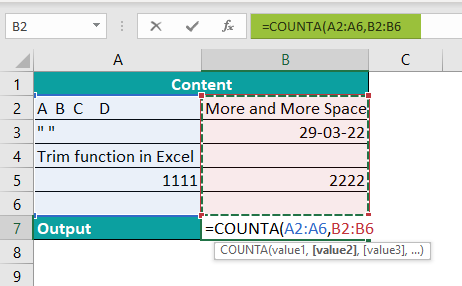Step 4: After entering the values in the preceding step, press the “Enter” key. The result is shown in cell B7 as ‘7’ in the image below.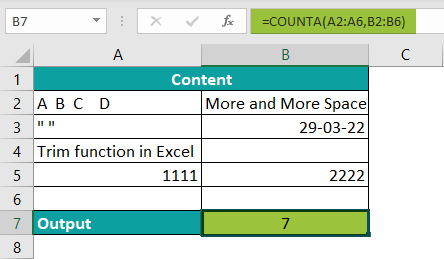Therefore, the COUNTA function counts all the numbers, letters, phrases, dates, and empty text strings within (“ “) quotes but does not count the blank spaces in the table. The total cell in the table is 10, but there are three empty cells in the table, which the COUNTA function did not count.